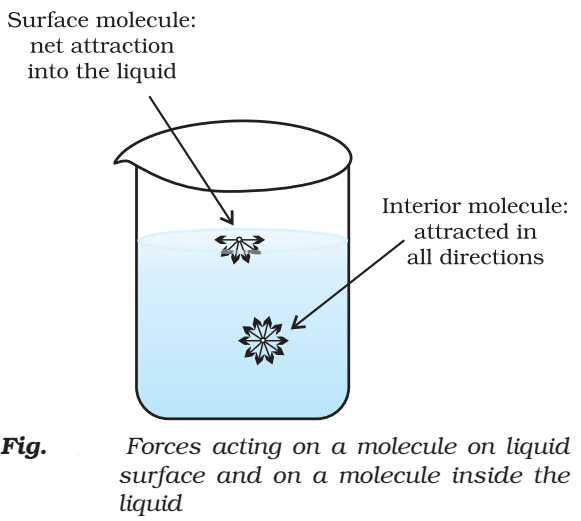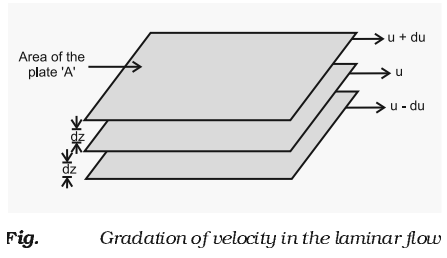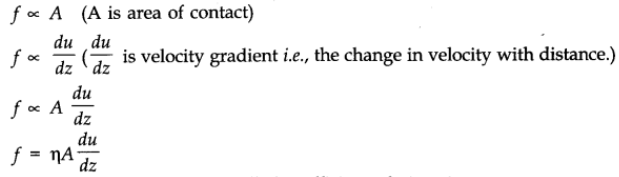Characteristics of Liquid State

• In liquid, intermolecular forces are strong in comparison to gas.
• They have definite volume but irregular shapes or we can say that they can take the shape of the container.
• Molecules of liquids are held together by attractive intermolecular forces.

# Vapour Pressure

The pressure exerted by the vapour of a liquid, at a particular temperature in a state of dynamic equilibrium, is called the vapour pressure.

Factor affecting vapour pressure:

Two important factors on which the vapour pressure of a liquids depends are:

• Nature of the liquid: If the intermolecular forces of attraction in the liquid are weak, the molecules can easily leave the liquid and come into the vapour phases and hence the vapour pressure is higher.
• Effect of temperature: As the temperature of a liquid is increased, the vapour pressure of the liquid increases.

# Boiling point

• Boiling point of a liquid is defined as the temperature at which the vapour pressure of the liquid becomes equal to the external pressure (i.e., the atmospheric pressure).
• When the external pressure is normal atmospheric pressure (i.e., 760 mm), the boiling point is called the normal boiling point.
• When the external pressure is equal to 1 bar, the boiling point is called standard boiling point of the liquid.

# Surface tension

It is the force acting per unit length perpendicular to the imaginary line drawn on the surface of liquid. It is denoted γ (gamma);

SI unit: Nm-1

Dimension: kgs-2The magnitude of surface tension of a liquid depends on the attractive forces between the molecules. It is measured with the help of an apparatus called stalagmometer.

Surface tension decreases as the temperature increases.

Rise of fall of liquid in a capacity tube is due to surface tension.

# Viscosity

• Viscosity is one of the characteristic properties of liquids.
• Viscosity is a measure of resistance to flow which arises due to the internal friction between layers of fluid as they slip past one another while liquid flows.• The type of flow in which there is a regular gradation of velocity in passing from one layer to the next is called laminar flow.
• The liquids which flow slowly have very high internal resistance, which is due to strong intermolecular forces and hence are said to be more viscous.
• When liquid flows, the layer immediately below it tries to related its flow while the one above tries to accelerate.

Thus, force is required to maintain the flow of layers.

This forceWhere ‘η’ is proportionality constant and is called coefficient of viscosity.

SI unit of viscosity coefficient = 1 newton second per square meter

= Ncm-2 = Pascal Second

In C.G.S system, the unit of coefficient of viscosity is poise.

1 poise = 1g cm-1 s-1

## Effect of temperature on viscosity:

Viscosity of liquids decreases as the temperature rises because at high temperature, molecules have high kinetic energy and can overcome the intermolecular forces to slip past one another.

Click on a star to rate it!

Average rating / 5. Vote count:

No votes so far! Be the first to rate this post.